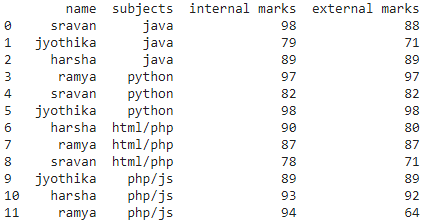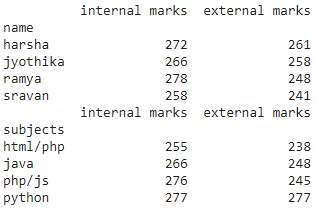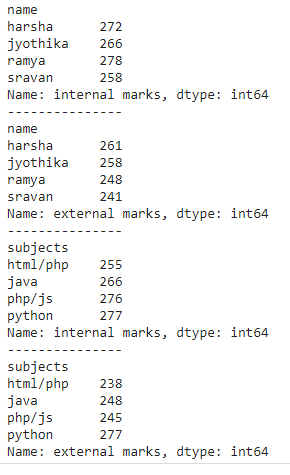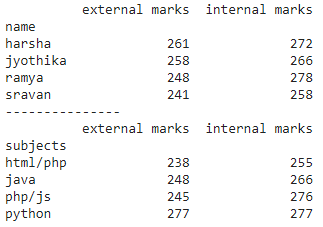How to Perform a SUMIF Function in Pandas?

• Last Updated : 22 Nov, 2021

sumif() function is used to perform sum operation by a group of items in the dataframe, It can be applied on single and multiple columns and we can also use this function with groupby function.

Method 1: SUMIF on all columns with groupby()

This function is used to display sum of all columns with respect to grouped column

Syntax: dataframe.groupby(‘group_column’).sum()

where

• dataframe is the input dataframe
• group_column is the column in dataframe to be grouped
• sum() function is to perform the sum operation

Python3

 # import pandas moduleimport pandas as pd  # create dataframe with 4 columnsdata = pd.DataFrame({      "name": ['sravan', 'jyothika', 'harsha',              'ramya', 'sravan', 'jyothika',              'harsha', 'ramya', 'sravan', 'jyothika',             'harsha', 'ramya'],    "subjects": ['java', 'java', 'java', 'python',                 'python', 'python', 'html/php',                  'html/php', 'html/php', 'php/js',                 'php/js', 'php/js'],    "internal marks": [98, 79, 89, 97, 82, 98, 90,                       87, 78, 89, 93, 94],    "external marks": [88, 71, 89, 97, 82, 98, 80,                       87, 71, 89, 92, 64],})  # display dataframeprint(data)

Output:Python3

 # import pandas moduleimport pandas as pd  # create dataframe with 4 columnsdata = pd.DataFrame({      "name": ['sravan', 'jyothika', 'harsha', 'ramya',             'sravan', 'jyothika', 'harsha', 'ramya',             'sravan', 'jyothika', 'harsha', 'ramya'],    "subjects": ['java', 'java', 'java', 'python',                 'python', 'python', 'html/php',                 'html/php', 'html/php', 'php/js',                 'php/js', 'php/js'],    "internal marks": [98, 79, 89, 97, 82, 98, 90,                       87, 78, 89, 93, 94],    "external marks": [88, 71, 89, 97, 82, 98, 80,                       87, 71, 89, 92, 64],})  # find sum of all columns group by nameprint(data.groupby('name').sum())    # find sum of all columns group by subjectsprint(data.groupby('subjects').sum())

Output:Method 2: SUMIF Function on One Column

Here we are performing sumif operation on one particular column by grouping it with one column

Syntax: dataframe.groupby(‘group_column’)[‘column_name].sum()

where

• dataframe is the input dataframe
• group_column is the column in dataframe to be grouped
• column_name is to get sum of this column with respect to grouped column
• sum() function is to perform the sum operation

Python3

 # import pandas moduleimport pandas as pd  # create dataframe with 4 columnsdata = pd.DataFrame({      "name": ['sravan', 'jyothika', 'harsha', 'ramya',             'sravan', 'jyothika', 'harsha', 'ramya',              'sravan', 'jyothika', 'harsha', 'ramya'],    "subjects": ['java', 'java', 'java', 'python',                 'python', 'python', 'html/php',                  'html/php', 'html/php', 'php/js',                 'php/js', 'php/js'],    "internal marks": [98, 79, 89, 97, 82, 98, 90,                       87, 78, 89, 93, 94],    "external marks": [88, 71, 89, 97, 82, 98, 80,                       87, 71, 89, 92, 64],})  # find sum of  columns group by# name with internal marks columnprint(data.groupby('name')['internal marks'].sum())  print("---------------")  # find sum of  columns group by# name with external marks columnprint(data.groupby('name')['external marks'].sum())  print("---------------")  # find sum of  columns group by# subjects with internal marks columnprint(data.groupby('subjects')['internal marks'].sum())  print("---------------")  # find sum of  columns group by# subjects with external marks columnprint(data.groupby('subjects')['external marks'].sum())

Output:Method 3: SUMIF Operation on multiple columns

Here we will use sumif operation on multiple columns.

Syntax: dataframe.groupby(‘group_column’)[[‘column_names’]].sum()

where,

• dataframe is the input dataframe
• group_column is the column in dataframe to be grouped
• column_names are to get sum of these columns with respect to grouped column
• sum() function is to perform the sum operation

Python3

 # import pandas moduleimport pandas as pd  # create dataframe with 4 columnsdata = pd.DataFrame({      "name": ['sravan', 'jyothika', 'harsha', 'ramya',             'sravan', 'jyothika', 'harsha', 'ramya',              'sravan', 'jyothika', 'harsha', 'ramya'],    "subjects": ['java', 'java', 'java', 'python',                 'python', 'python', 'html/php',                  'html/php', 'html/php', 'php/js',                  'php/js', 'php/js'],    "internal marks": [98, 79, 89, 97, 82, 98, 90,                       87, 78, 89, 93, 94],    "external marks": [88, 71, 89, 97, 82, 98, 80,                       87, 71, 89, 92, 64],})  # find sum of  columns group by name with# external marks and internal marks columnprint(data.groupby('name')[['external marks',                            'internal marks']].sum())  print("---------------")  # find sum of  columns group by subjects# with external marks and internal marks columnprint(data.groupby('subjects')[['external marks',                                'internal marks']].sum())

Output:My Personal Notes arrow_drop_up